# Basic Circuit Troubleshooting

## Basic Electricity

• #### Question 1

Determine if the light bulb will de-energize for each of the following breaks in the circuit. Consider just one break at a time: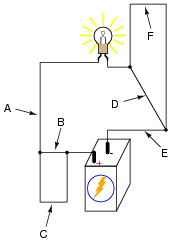Choose one option for each point:
• A: de-energize / no effect
• B: de-energize / no effect
• C: de-energize / no effect
• D: de-energize / no effect
• E: de-energize / no effect
• F: de-energize / no effect
• #### Question 2

Examine the following illustration of a simple battery-switch-lamp circuit, connected together using screw-terminal blocks, each connection point on each terminal block identified by a unique number: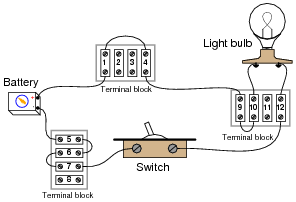Determine whether or not voltage should be present between the following pairs of terminal block points with the switch in the ON position:

• Points 1 and 5:
• Points 6 and 7:
• Points 4 and 10:
• Points 9 and 12:
• Points 6 and 12:
• Points 9 and 10:
• Points 4 and 7:

Now, determine whether or not voltage should be present between the following pairs of terminal block points with the switch in the OFF position:

• Points 1 and 5:
• Points 6 and 7:
• Points 4 and 10:
• Points 9 and 12:
• Points 6 and 12:
• Points 9 and 10:
• Points 4 and 7:
• #### Question 3

The following battery-switch-lamp circuit has a problem. Over time, corrosion has developed between the wire end and the screw terminal labeled “4” on the upper side of the top terminal block. This corroded connection now has a high resistance instead of a low resistance as it should. As a result, the light bulb does not energize when the switch is turned ON: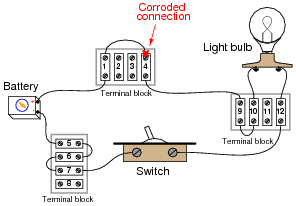Taking voltage measurements with a voltmeter, how do you think this corrosion problem will reveal itself with the switch on the ON position? In other words, which voltage measurement in this circuit (i.e. between which pair or pairs of terminal block points) will be unusual as a result of the bad connection at point #4, and approximately how much voltage should that measurement be?

• #### Question 4

In this battery-switch-lamp circuit, the metal filament wire inside the lamp has burned up, so that it no longer forms an electrically continuous connection. In other words, the filament has failed “open.”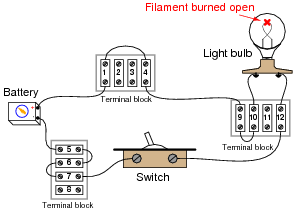Of course, this means the lamp will not turn on, no matter what is done with the switch. It also means that most of the voltage measurements taken in the circuit will be the same as with a properly operating circuit. There is, however, one voltage measurement which will be different in the circuit with the burned-out filament than in a properly working circuit. Identify what pair or pairs of terminal block points this different voltage will be measured between, what switch state (ON or OFF) it will appear in, and what this different voltage measurement will actually be relative to the battery voltage.

• #### Question 5

In this circuit, where would you expect to measure full battery voltage (between what pairs of test points)?• #### Question 6

In this circuit, where would you not expect to measure significant voltage (between what pairs of test points)?• #### Question 7

Suppose this battery and light bulb circuit failed to work. Using nothing but a voltmeter, how would you check the circuit to determine where the problem is located? Note: the letters indicate “test points” along the wiring where you may probe with the circuit with your voltmeter.• #### Question 8

Suppose this battery and light bulb circuit failed to work:Using a voltmeter, a technician measures full battery voltage between the points C and H. What does this single measurement indicate about the condition of the circuit? Be as specific as you can.

• #### Question 9

Suppose this battery and light bulb circuit failed to work:Using a voltmeter, a technician measures full battery voltage between the points C and H. The result of this single measurement indicates which half of the circuit there is a definite problem in. What would you recommend as the next voltmeter measurement to take in troubleshooting the circuit, following the same “divide in half” strategy?

• #### Question 10

Suppose this battery and light bulb circuit failed to work:Using a voltmeter, a technician measures 0 volts between the points C and H. What does this single measurement indicate about the condition of the circuit? Be as specific as you can.

• #### Question 11

Suppose this battery and light bulb circuit failed to work:Using a voltmeter, a technician measures 0 volts between the points C and H. The result of this single measurement indicates which half of the circuit there is a definite problem in. What would you recommend as the next voltmeter measurement to take in troubleshooting the circuit, following the same “divide in half” strategy?

• #### Question 12

Suppose this battery and light bulb circuit failed to work:Using nothing but a voltmeter, a technician measures voltage between the following sets of points:

• Between A and C: 0 volts
• Between D and G: 12 volts
• Between E and J: 0 volts
• Between B and E: 12 volts

From these voltage measurements, what can you tell about the condition of the battery, wiring, and light bulb? Be as specific as you can.

Challenge question: identify which of the four measurement are unnecessary in determining the precise location of the fault in this circuit.

• #### Question 13

The circuit shown here is called a “bridge rectifier,” and its purpose is to convert alternating current (from the “power-supply” unit) into direct current. Suppose you were instructed to check the continuity of the switch (SW1) mounted on the printed circuit board. What would be a fast and effective way of testing this switch’s continuity (ideally, without removing the switch from the circuit board)?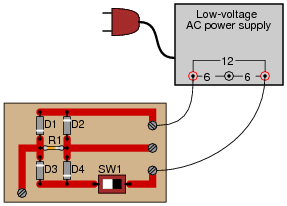• #### Question 14

Identify which of these are true statements:

1. Between two points that are electrically common to each other, there is guaranteed to be zero voltage.
2. If zero voltage is measured between two points, those points must be electrically common to each other.
3. Between two points that are not electrically common to each other, there is guaranteed to be voltage.
4. If voltage is measured between two points, those points must not be electrically common to each other.

• #### Question 15

Suppose a technician were troubleshooting the following circuit, whose light bulb refused to light up: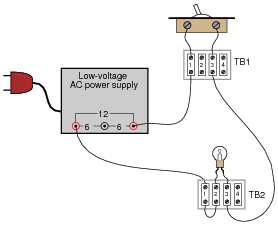The technician records their steps on a piece of paper divided into two columns: Observations, and Conclusions, drawing a horizontal line underneath each conclusion after it is made:Critique this technician’s troubleshooting job, noting any errors or unnecessary steps.

• #### Question 16

The following circuit has a problem. When the switch is closed, the lamp does not turn on:Identify which of these hypothetical faults could account for this problem, and which could not account for the problem. In other words, which of these faults are possible, and which are not possible, given the symptoms exhibited by the circuit? Consider each of these hypothetical faults one at a time (no multiple, simultaneous faults):

• Light bulb filament failed open
• Switch failed shorted
• Switch failed open
• #### Question 17

The following circuit has a problem. Switch #1 is able to control lamp #1, but lamp #2 never comes on no matter what is done with switch #2:Identify which of these hypothetical faults could account for this problem, and which could not account for the problem. In other words, which of these faults are possible, and which are not possible, given the symptoms exhibited by the circuit? Consider each of these hypothetical faults one at a time (no multiple, simultaneous faults):

• Switch #2 failed open
• Switch #2 failed shorted
• Switch #1 failed open
• Switch #1 failed shorted
• Open wire between test points 1 and 2 (between TP1 and TP2)
• Open wire between test points 5 and 6 (between TP5 and TP6)
• #### Question 18

The following circuit has a problem. Switch #2 is able to control lamp #2, but lamp #1 never comes on no matter what is done with switch #1:Identify which of these hypothetical faults could account for this problem, and which could not account for the problem. In other words, which of these faults are possible, and which are not possible, given the symptoms exhibited by the circuit? Consider each of these hypothetical faults one at a time (no multiple, simultaneous faults):

• Lamp #1 filament failed open
• Lamp #2 filament failed open
• Switch #2 failed open
• Switch #2 failed shorted
• Switch #1 failed open
• Switch #1 failed shorted
• Open wire between test points 1 and 2 (between TP1 and TP2)
• Open wire between test points 4 and 5 (between TP4 and TP5)
• Open wire between test points 5 and 6 (between TP5 and TP6)

### Related Content

Published under the terms and conditions of the Creative Commons Attribution License•Bins July 14, 2022
•pnwinside February 23, 2023